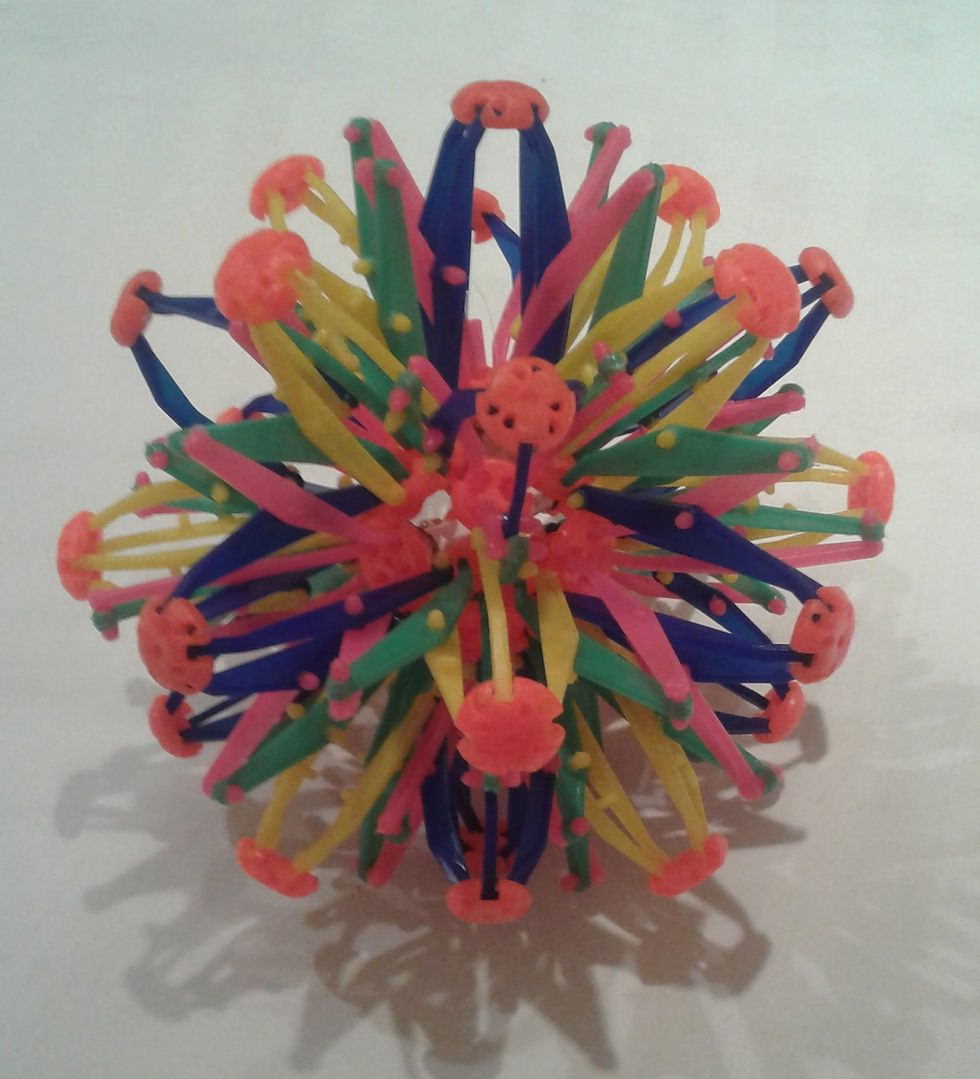top of page
Search

# 26 pointed stars sacred geometry encoding the seed of life and 64 tetrahedron grid

Updated: Apr 11, 2021

This 3D star is comprised of 26 points:It has 26 joints on the outside and 26 joints on the inside and when expanded it has 24 square like faces, 24+26+26=76=38+38

The 26 joints on the inside form a sphere making 27 and 27(142857(enneagram)/124875(fingerprint of god)=3 cubes=18=star tetrahedron/seed of life and 142857 is formed by 1/7 and represents the 7 sephiroth of construction and form the hexagon that is in the star tetrahedron which constructs the seed of life, they also form the 7 yods in the tetractys with the other 3 being 369 Also 142857=(3^3)×11×13×37

Also the 26 pointed stars when expanded form a bigger sphere which is a spherical cube that is 3×3×3, also 3 tree of life's=27+1=28

There are 26 superstring dimensions which corresponds to this 26 pointed star and the last line of the tetragrammaton/tetractys also the equation which produces -1/12(infinite tetrahedron grid/infinite tetractys/7 tetrahedral tetractys) from the 26 superstring dimensions, minuses 2 from the number of dimensions so 26-2=24 which is the number of faces and 24=4 cubes(444)=64 tetrahedron grid also 24=12+12=2 dodecahedron and as we know the dodecahedron is the star tetrahedron mathematical and as we know 2 star tetrahedron form the fruit of life which encoded metatrons cube/64 tetrahedron grid.

26=13+13 and as we know 13 is the star tetrahedron tetractys/fruit of life this is interesting because it shows a star tetrahedron tetractys encoded in a star tetrahedron tetractys or a 64 tetrahedron grid making up a bigger tetrahedron grid aka the 512 tetrahedron grid which encodes the Trinity 26 dimensions and the 12 vibrational dimension also the star tetrahedron tetractys is encoded in the seed of life.

Also the 26 pointed star as we know is equal to the last line of the tetragrammaton and that line also encodes the fine structure constant which is related to light and this shows this structure can be formed by the process in quantum atom theory and this structure encodes the 64 tetrahedron grid/E8 lie group which is formed by the same process!

This post is also talked about in the new blog on the wiki.